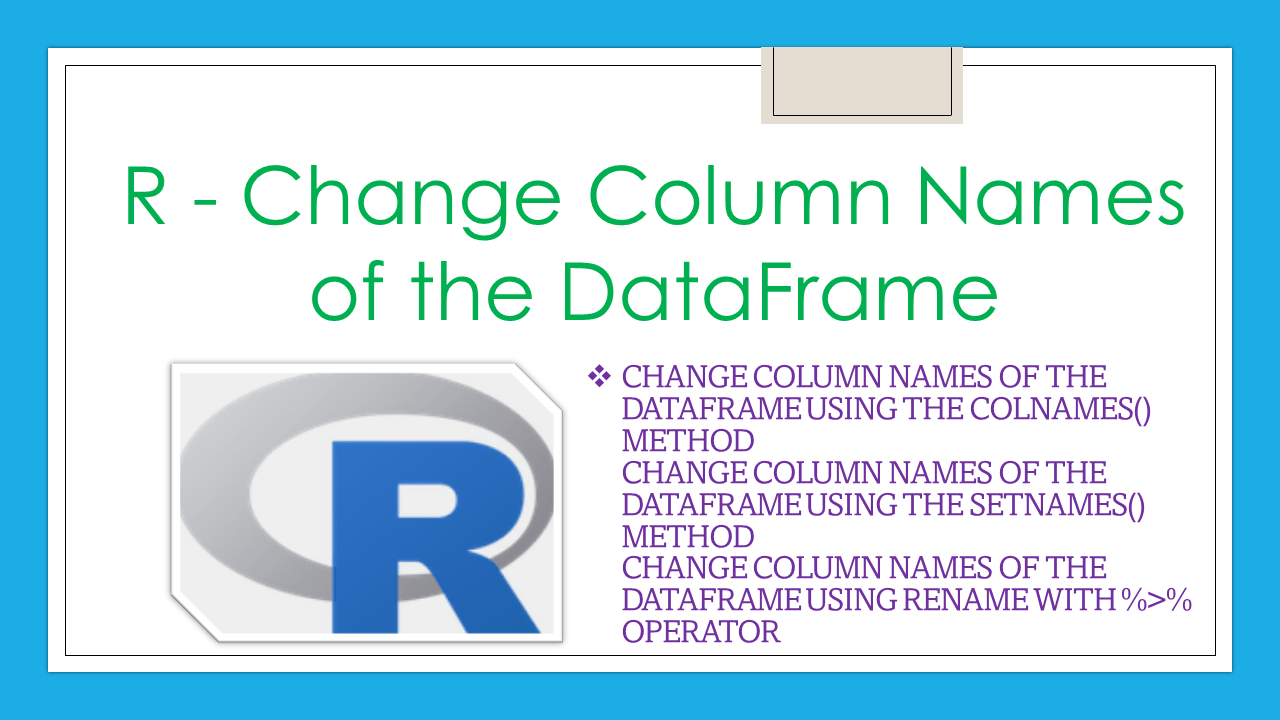# Changing Column Names in R

How to change the column names in RDataFrame (data.frame). Changing the column names in R is pretty easy, you can do it in three methods. We can able to change single or all column names using colnames(). Next, we are using setNames() which is used to change all the column names in a dataframe. Finally, we are using rename() method with the `%>% operator` to change all the column names.

## 1 Quick Examples of Changing Column Names

Following are quick examples of changing column names in R DataFrame.

``````# Create dataframe
#change the column names to c1,c2,c3,c4 and c5 using colnames()
colnames(my_dataframe) =  c('c1','c2','c3','c4','c5')

#change second column to c2
colnames(my_dataframe) ="c2"

#change fifth column to c5
colnames(my_dataframe) ="c5"

#change the column names to c1,c2,c3,c4 and c5 using setNames()
print(setNames(my_dataframe, c('c1','c2','c3','c4','c5')))

library("dplyr")

#change the column names to c1,c2,c3,c4 and c5 using rename()
print(my_dataframe %>% rename(c1 = id,c2 = pages,c3 = name,c4 = chapters,c5 = price))
``````

Let’s create the dataframe with five rows and five columns.

``````
# Create dataframe
my_dataframe=data.frame(id=c(11,22,33,44,55),
pages=c(32,45,33,22,56),
name=c("spark","python","R","java","jsp"),
chapters=c(76,86,11,15,7),
price=c(144,553,321,567,890))

# Display the dataframe
print(my_dataframe)
``````

Output:

We can see that the column names are: `id`, `pages`, `name`, `chapters`, and `price`. Let’s change the column names for this dataframe.

## 2. Changing Column Names in R using colnames()

colnames() is the method available in R that is used to change all column names present in the dataframe. It takes

Syntax:

``````#Syntax for colnames to rename all columns
colnames(my_dataframe) =  c('new_column_name',...........)
``````

where,

1. `my_dataframe` is the input dataframe
2. `new_column_name` is the new column name that replaces the old column name

Example:

Let’s change the column names of the above dataframe to `c1`,`c2`,`c3`,`c4`, and `c5`.

``````
#Change the column names to c1,c2,c3,c4 and c5 using colnames()
colnames(my_dataframe) =  c('c1','c2','c3','c4','c5')

#Display the dataframe
print(my_dataframe)
``````

Output:

``````
c1 c2     c3 c4  c5
1 11 32  spark 76 144
2 22 45 python 86 553
3 33 33      R 11 321
4 44 22   java 15 567
5 55 56    jsp  7 890
``````

We can see that column names are changed.

If we want to change a particular column by index, then we have to specify the index of the column. In R, indexing starts with 1.

Syntax:

``````#Syntax to rename single column wth colnames() by column index.
colnames(my_dataframe)[index_pos] ="new_column_name"
``````

where `index_pos` represent the column index.

Example:

In this example, we will change the second column to `c2` and the fifth column to `c5`.

``````# Create dataframe
my_dataframe=data.frame(id=c(11,22,33,44,55),
pages=c(32,45,33,22,56),
name=c("spark","python","R","java","jsp"),
chapters=c(76,86,11,15,7),
price=c(144,553,321,567,890))

#change second column to c2
colnames(my_dataframe) ="c2"

#change fifth column to c5
colnames(my_dataframe) ="c5"

#display the dataframe
print(my_dataframe)
``````

Output:

``````
id c2   name chapters  c5
1 11 32  spark       76 144
2 22 45 python       86 553
3 33 33      R       11 321
4 44 22   java       15 567
5 55 56    jsp        7 890
``````

We can see that the second and fifth column names are changed.

## 3. Change Column Names using setNames()

setNames() is the method available in R, which is used to change all the columns present in the dataframe.

Syntax:

``````#Syntax for setNames() to change the column names
setNames(my_dataframe, c('new_column_name',...........))
``````

where,

1. my_dataframe is the input dataframe
2. new_column_name is the new column name that replaces the old column name

Example:

Let’s change the columns of the above dataframe to c1,c2,c3,c4, and c5.

``````# Create dataframe
my_dataframe=data.frame(id=c(11,22,33,44,55),
pages=c(32,45,33,22,56),
name=c("spark","python","R","java","jsp"),
chapters=c(76,86,11,15,7),
price=c(144,553,321,567,890))

# Change the column names to c1,c2,c3,c4 and c5 using setNames()
print(setNames(my_dataframe, c('c1','c2','c3','c4','c5')))
``````

Output:

``````
c1 c2     c3 c4  c5
1 11 32  spark 76 144
2 22 45 python 86 553
3 33 33      R 11 321
4 44 22   java 15 567
5 55 56    jsp  7 890
``````

We can see that column names are changed.

## 4. Change Column Names using dplyr rename()

rename() is the method available in the dplyr package, which is used to change the particular columns present in the dataframe. The operator %>% is used to load the renamed column names to the dataframe. At a time it will change single or multiple column names. But we have to load the dplyr library in order to use this method.

Syntax:

``````#Syntax to load the dplyr library
library("dplyr")
#Syntax to change column names using rename()
my_dataframe %>% rename(new_column_name = old_column_name,...........)
``````

where,

1. my_dataframe is the input dataframe
2. new_column_name is the new column name that replaces the old_column_name

Example:

Let’s change the columns of the above dataframe to c1,c2,c3,c4, and c5.

``````#Load the dplyr library
library("dplyr")

#Change the column names to c1,c2,c3,c4 and c5 using rename()
print(my_dataframe %>% rename(c1 = id,c2 = pages,c3 = name,c4 = chapters,c5 = price))
``````

Output:

``````
c1 c2     c3 c4  c5
1 11 32  spark 76 144
2 22 45 python 86 553
3 33 33      R 11 321
4 44 22   java 15 567
5 55 56    jsp  7 890
``````

We can see that column names are changed.

## Conclusion

In this article, we have seen different ways of changing the column names in R, using colnames(), rename(), and setNames() methods. To change single or multiple columns use colnames() and rename() methods.

## References

### Naveen (NNK)

Naveen (NNK) is a Data Engineer with 20+ years of experience in transforming data into actionable insights. Over the years, He has honed his expertise in designing, implementing, and maintaining data pipelines with frameworks like Apache Spark, PySpark, Pandas, R, Hive and Machine Learning. Naveen journey in the field of data engineering has been a continuous learning, innovation, and a strong commitment to data integrity. In this blog, he shares his experiences with the data as he come across. Follow Naveen @ @ LinkedIn